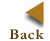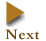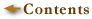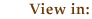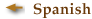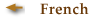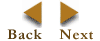1. Basic Terminology

Key Concepts

digital images
resolution
pixel dimensions
bit depth
dynamic range
file size
compression
file formats

FILE SIZE is calculated by multiplying the surface area of a document (height x width) to be scanned by the bit depth and the dpi2. Because image file size is represented in bytes, which are made up of 8 bits, divide this figure by 8.

 Formula 1 for File Size File Size = (height x width x bit depth x dpi2) / 8

If the pixel dimensions are given, multiply them by each other and the bit depth to determine the number of bits in an image file. For instance, if a 24-bit image is captured with a digital camera with pixel dimensions of 2,048 x 3,072, then the file size equals (2048 x 3072 x 24)/8, or 18,874,368 bytes.

 Formula 2 for File Size File Size = (pixel dimensions x bit depth) / 8

File size naming convention: Because digital images often result in very large files, the number of bytes is usually represented in increments of 210 (1,024) or more:

1 Kilobyte (KB) = 1,024 bytes
1 Megabyte (MB) = 1,024 KB
1 Gigabyte (GB) = 1,024 MB
1 Terabyte (TB) = 1,024 GB
 Reality Check What is the file size for a US letter-size page captured bitonally at 100 dpi? bytes

© 2000-2003 Cornell University Library/Research Department